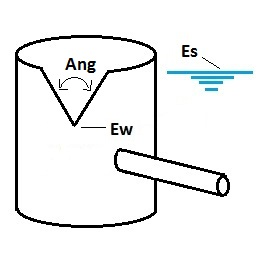* Log In to use the Calculate function * Become a Member!

Calculate The Discharge of V-notch Weir for Weir Outlet Control With Free Discharge:

Calculation:
Designer/Checker:

Input:(Note: All input Metric units are converted to English units for the equations below and then the output English units are converted back to Metric)

Angle of V-notch (Ang - degrees)
Water surface elevation at design volume (Es - ft or m)
Elevation of vertex of V-notch weir (Ew - ft or m)
Weir coefficient (Cw)

Typical values for Cw (English Units):
V-Notch Weir: Cw = 2.5

Output: